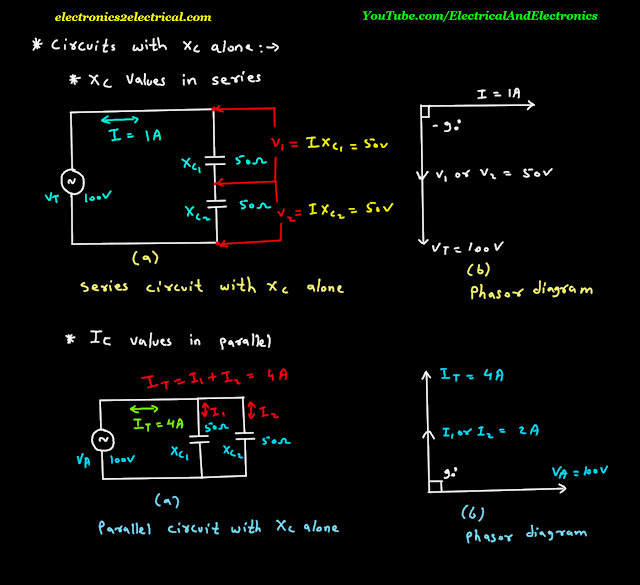0 like 0 dislike

Circuits with capacitive reactance X C alone.

Capacitive reactance X C values in series.

There is no resistance or inductive reactance only capacitive reactance is there.

Let two capacitive reactances are connected in series.

The 100 volts A C voltage is applied.

The voltage across capacitive reactance X C 1 is V 1 is equal to Current I into X C 1.

The voltage across capacitive reactance X C 2 is V 2 is equal to current into X C 2.

The current I can be find by Ohm's law.

The phasor diagram shows series current I horizontal because it is taken as reference phasor.

Series current I is taken as reference phasor because same current flowing through both the capacitive reactances.

The voltage across capacitive reactance lags the capacitive charge and discharge current I by 90 degree.Now capacitive reactances in parallel.

Capacitive current I C values in parallel.

Let two capacitive reactances are connected in parallel.

100 volts A C voltage source is applied.

The current I T can be find by Ohm's law.

The total current I T is equal to I 1 plus I 2.

The current I 1 is equal to voltage V A upon X C 1.

Current I 2 is equal to voltage V A upon X C 2.

The phasor diagram shows the voltage V A as reference phasor.

Voltage V A is common voltage to both X C 1 and X C 2 hence it is taken as reference phasor and horizontal in phasor diagram.

The total current I T leads voltage V A by 90 degree.

edited